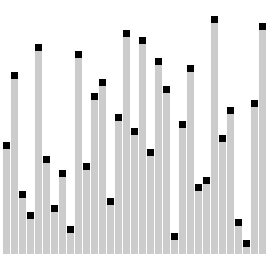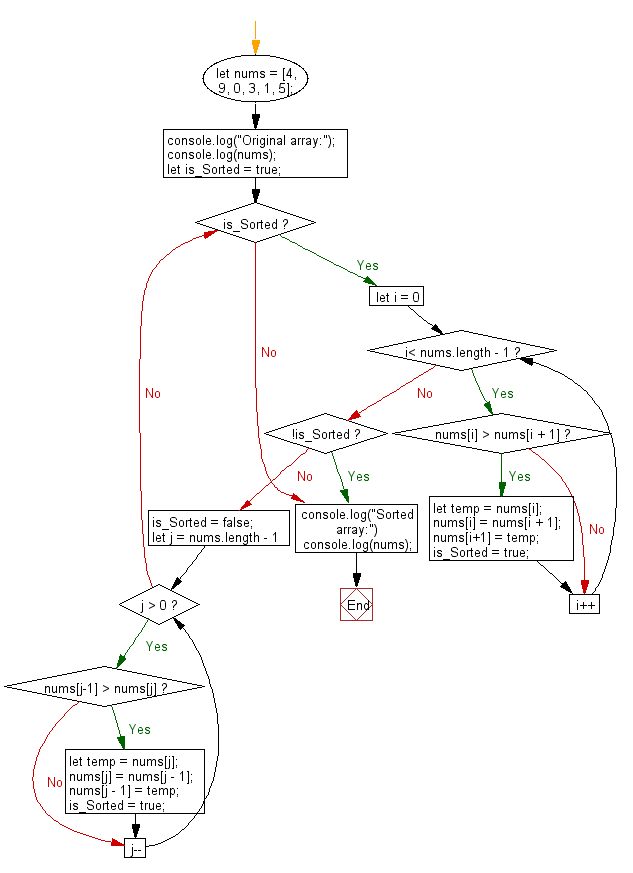﻿ PHP: Sort a list of elements using Comb sort - w3resource

# PHP Searching and Sorting Algorithm: Comb sort

## PHP Searching and Sorting Algorithm: Exercise-8 with Solution

Write a PHP program to sort a list of elements using Comb sort.

The Comb Sort is a variant of the Bubble Sort. Like the Shell sort, the Comb Sort increases the gap used in comparisons and exchanges. Some implementations use the insertion sort once the gap is less than a certain amount. The basic idea is to eliminate turtles, or small values near the end of the list, since in a bubble sort these slow the sorting down tremendously. Rabbits, large values around the beginning of the list do not pose a problem in bubble sort.
In bubble sort, when any two elements are compared, they always have a gap of 1. The basic idea of comb sort is that the gap can be much more than 1.

Visualization of comb sort :Animation credits : Jerejesse

Sample Solution :

PHP Code :

``````<?php
function combSort(\$my_array){
\$gap = count(\$my_array);
\$swap = true;
while (\$gap > 1 || \$swap){
if(\$gap > 1) \$gap /= 1.25;
\$swap = false;
\$i = 0;
while(\$i+\$gap < count(\$my_array)){
if(\$my_array[\$i] > \$my_array[\$i+\$gap]){
list(\$my_array[\$i], \$my_array[\$i+\$gap]) = array(\$my_array[\$i+\$gap],\$my_array[\$i]);
\$swap = true;
}
\$i++;
}
}
return \$my_array;
}
\$test_array = array(3, 0, 2, 5, -1, 4, 1);
echo "Original Array :\n";
echo implode(', ',\$test_array );
echo "\nSorted Array\n:";
echo implode(', ',combSort(\$test_array)). PHP_EOL;
?>
```
```

Sample Output:

```Original Array :
3, 0, 2, 5, -1, 4, 1
Sorted Array :
-1, 0, 1, 2, 3, 4, 5
```

Flowchart :PHP Code Editor:

Have another way to solve this solution? Contribute your code (and comments) through Disqus.

What is the difficulty level of this exercise?

Test your Programming skills with w3resource's quiz.

﻿